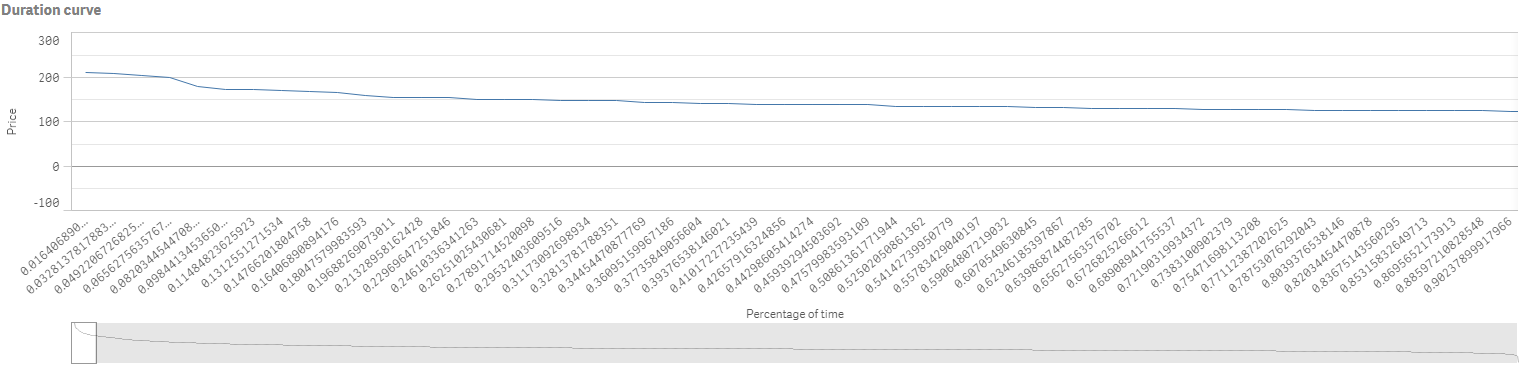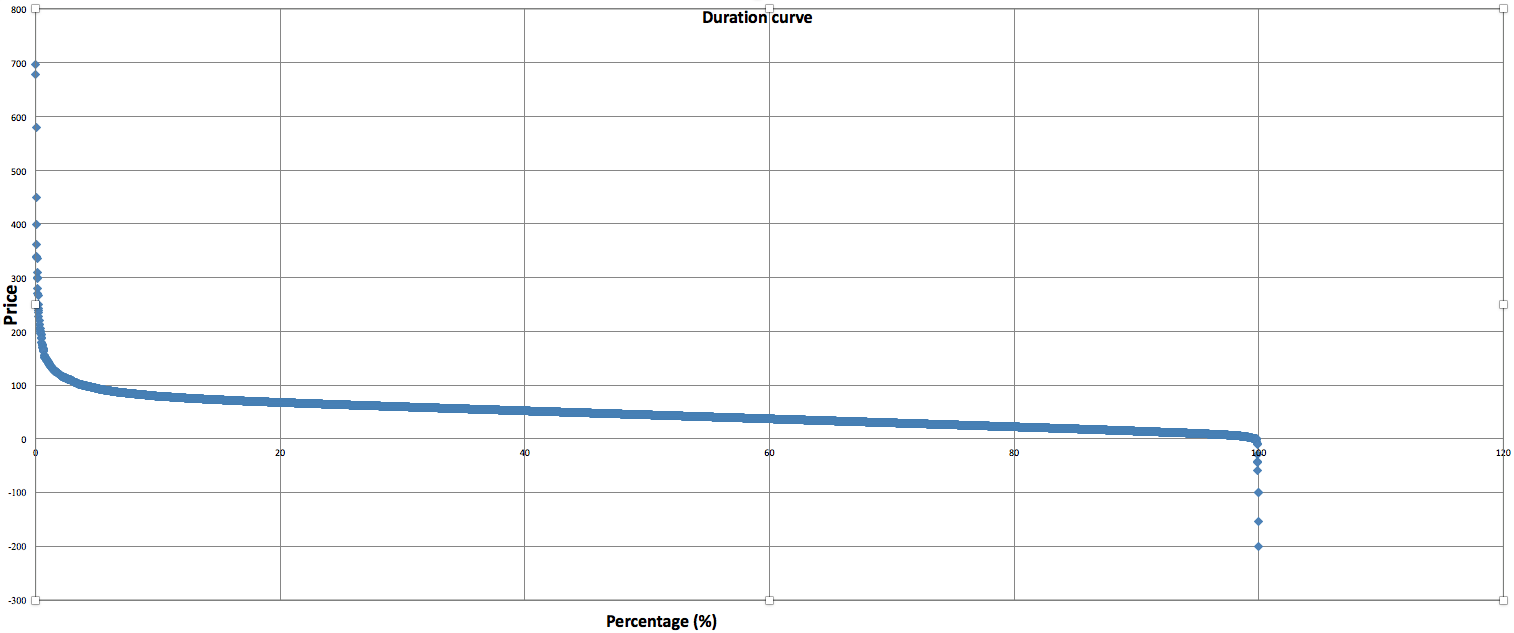# QlikView App Dev

Discussion Board for collaboration related to QlikView App Development.

cancel
Showing results for
Did you mean:Not applicable

## Change x-axis scale

Hi,

I am a very new user of Qlik Sense. I encounter some issues with the visualisation of a line chart. So I've computed the following line chart :Dimension : 100*aggr(Rank(Price,1,1),date)/(max(total aggr(rank(Price),date))+1)

Measure : Price

And I would like something more similar to this (It is the same graph computed on Excel)  :The general trend seems more clear in the second chart. So I wanted to know how to get a more similar chart in qlik (maybe change the scale of the X-axis and only represent integer value would reduce the length of the first graph)

1 Solution

Accepted SolutionsPartner

And sorry for mistake

if(PercentField >0.00 and PercentField<=0.20,'20',

if(PercentField >0.20 and PercentField<=0.40,'40',

if(PercentField >0.40 and PercentField<=0.60,'60',

if(PercentField >0.60 and PercentField<=0.80,'80',

if(PercentField >0.80 and PercentField<=1,'100',

if(PercentField =0.00 ,'0')))))) as NewField

8 RepliesMVP

Can you provide QVF file?

Before develop something, think If placed (The Right information | To the right people | At the Right time | In the Right place | With the Right context)Champion III

May be try using scatter or grid chart.Not applicable
Author

Sorry I am not sure to be able to provide you the QVF file but here's an excel with the data regarding to my question (the dimension and measure of the line chart are mentioned in my first post)Not applicable
Author

Hi, anyone knows how to resolve my problem ?Partner

Create a Dimension by Using Class(Fieldname*100,20)

Else in Script,

if(PercentField >0.00 and PercentField<=0.20,'20',

if(PercentField >0.20 and PercentField<=0.40,'40',

if(PercentField >0.40 and PercentField<=0.60,'60',

if(PercentField >0.60 and PercentField<=0.80,'80',

if(PercentField >0.80 and PercentField<=1,'100',

if(PercentField =0.80 ,'0')))))) as NewField

Use this new field in DimensionNot applicable
Author

Hi, I didn't get this condition : if(PercentField =0.80 ,'0'). Could you give me more explanation please ?Partner

Here you are comparing Values of Fields to create buckets shown in the chart created in Excel..Partner

And sorry for mistake

if(PercentField >0.00 and PercentField<=0.20,'20',

if(PercentField >0.20 and PercentField<=0.40,'40',

if(PercentField >0.40 and PercentField<=0.60,'60',

if(PercentField >0.60 and PercentField<=0.80,'80',

if(PercentField >0.80 and PercentField<=1,'100',

if(PercentField =0.00 ,'0')))))) as NewField